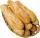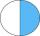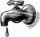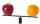# Paper collecting

At the paper collecting contest gathered Franta 2/9 ton, Karel 1/4 ton and Patrick 19/36 tons of paper. Who have gathered the most and the least (x, y - write down the weights in tonnes).

Result

x =  0.543 t
y =  0.222 t

#### Solution:Leave us a comment of example and its solution (i.e. if it is still somewhat unclear...):

Showing 0 comments:Be the first to comment!#### To solve this example are needed these knowledge from mathematics:

Need help calculate sum, simplify or multiply fractions? Try our fraction calculator.

## Next similar examples:

1. ZdeněkZdeněk picked up 15 l of water from a 100-liter full-water barrel. Write a fraction of what part of Zdeněk's water he picked.
2. CakesOn the bowl were a few cakes. Jane ate one-third of them, Dana ate a quarter of those cakes that remained. a) What part (of the original number of cakes) Dana ate? b) At least how many cakes could be (initially) on thebowl?
3. Fraction to decimalWrite the fraction 3/22 as a decimal.
4. Mixed2improperWrite the mixed number as an improper fraction. 166 2/3
5. In fractionsAn ant climbs 2/5 of the pole on the first hour and climbs 1/4 of the pole on the next hour. What part of the pole does the ant climb in two hours?
6. Lengths of the poolMiguel swam 6 lengths of the pool. Mat swam 3 times as far as Miguel. Lionel swam 1/3 as far as Miguel. How many lengths did mat swim?
7. RollsMom bought 13 rolls. Dad ate 3.5 rolls. How many rolls left when Peter yet put two at dinner?
8. Reduce to lowest termsReduce to lowest terms : 32/124
9. Fraction and a decimalWrite as a fraction and a decimal. One and two plus three and five hundredths
10. BuingBrother got to buy 240 CZK and could buy for 1/8 what he wanted. Could he pay the rest of the purchase for 200 CZK?
11. DenominatorCalculate the missing denominator x: ?
12. Comparing and sortingArrange in descending order this fractions: 2/7, 7/10 & 1/2
13. Adding mixed numbersAdd this two mixed numbers: 1 5/6 + 2 2/11=
14. The tapFor one day flows 148 l of water out of the tap. How much water will flow out for 3/4 day?
15. SchoolThere are 150 pupils in grade 5 . 2/3 of it are female. By what fractions are the males?
16. CompareCompare with characters >, <, =: 85.57 ? 80.83
17. Addition of Roman numbersAdded together and write as decimal number: LXVII + MLXIV